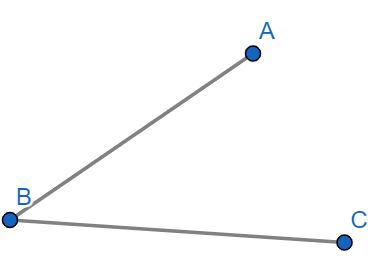Courses
Courses for Kids
Free study material
Offline Centres
MoreLast updated date: 08th Dec 2023
Total views: 281.1k
Views today: 2.81k

# Illustrate, if possible, the following with a rough diagram. A polygon with two sidesVerified
281.1k+ views
Hint: We know that a polygon is a closed figure. So in order to draw a polygon with two sides, we must check out if we can draw a figure formed with two sides. in order to draw a closed figure, the minimum requirement of sides is three.

Complete step-by-step solution:
Now let us briefly consider a polygon. Polygon is derived from the greek language in which poly means “many” and gon means “angle”. A polygon is a two dimensional figure which is formed by a finite number of line segments. If a figure includes a curve, then it is not considered as a polygon. If all the sides of the polygon are equal and has equal angles, then it is considered to be a regular polygon else if an irregular polygon. There are two types of polygons. They are: convex polygon and a concave polygon. A polygon that intersects itself is called a complex polygon.
Now let us try if we can draw a polygon with two sides.
We know that the minimum requirement of sides to form a polygon is three.
So we can conclude that we cannot form a polygon with two sides.
When we try to draw with two sides, we will be able to form an angle or a line segment with segments in it.Note: We can never form a polygon with one or two sides because the polygon should be a closed figure. With the help of one or two sides, we will not be able to form a polygon which is closed. We can also say that the number of sides can be from three to the required number of sides.